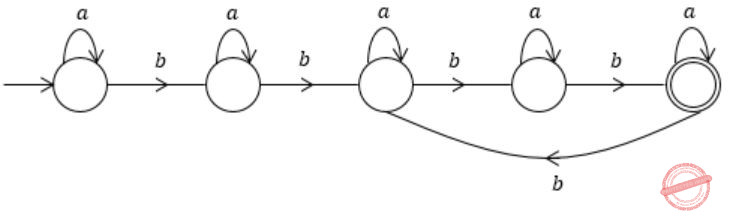## Regular Languages

 Question 1

Which one of the following is TRUE?

 A The language L={an bn│n≥0} is regular. B The language L={an│n is prime} is regular. C The language L={w│w has 3k+1b's for some k∈N with Σ={a,b} } is regular. D The language L={ww│w∈Σ* with Σ={0,1} } is regular.
Theory-of-Computation       Regular Languages       GATE 2014 [Set-1]
Question 1 Explanation:
The Language L= {an bn | n>=0} is CFL but not regular, as it requires comparison between a’s and b’s.
L = {an | n is prime} is CSL, as calculation of “n is prime” can be done by LBA (Turing machine)
L = {ww | w ∈ ∑*} is CSL.
But L = { w | w has 3k+1 b’s for some k ∈ natural number} is regular.
Lets take values of k={1,2,3,….}
So number of b’s will be {4, 7, 10,……….} and number of a’s can be anything.
The DFA will beQuestion 2

If L1 = {an|n≥0} and L2 = {bn|n≥0}, consider

(I) L1·L2 is a regular language
(II) L1·L2 = {anbn|n≥0}

Which one of the following is CORRECT?

 A Only (I) B Only (II) C Both (I) and (II) D Neither (I) nor (II)
Theory-of-Computation       Regular Languages       GATE 2014 [Set-2]
Question 2 Explanation:
The regular expression equivalent to L1 and L2 are a* and b* respectively.
Since L1 and L2 both are regular languages and regular languages are closed under concatenation. So their concatenation (i.e., L1⋅ L2) must also be a regular language.
So, L1⋅L2 = { anbn | n ≥ 0}
Hence, statement (i) is True but statement (ii) is False.
There are 2 questions to complete.

Register Now